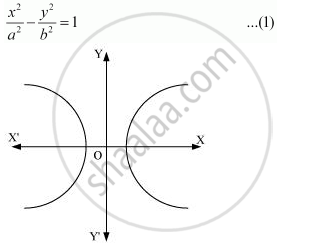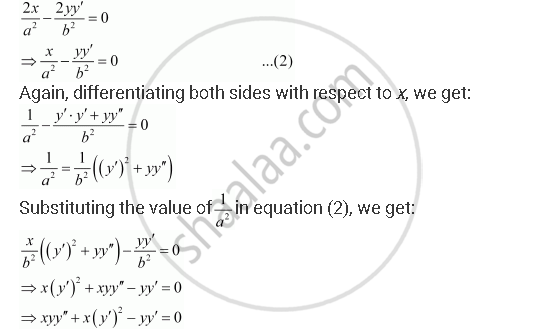Share

# Form the Differential Equation of the Family of Hyperbolas Having Foci on X-axis and Centre at Origin. - CBSE (Science) Class 12 - Mathematics

ConceptProcedure to Form a Differential Equation that Will Represent a Given Family of Curves

#### Question

Form the differential equation of the family of hyperbolas having foci on x-axis and centre at origin.

#### Solution

The equation of the family of hyperbolas with the centre at origin and foci along the x-axis is:Differentiating both sides of equation (1) with respect to x, we get:This is the required differential equation.

Is there an error in this question or solution?

#### APPEARS IN

NCERT Solution for Mathematics Textbook for Class 12 (2018 to Current)
Chapter 9: Differential Equations
Q: 9 | Page no. 391
Solution Form the Differential Equation of the Family of Hyperbolas Having Foci on X-axis and Centre at Origin. Concept: Procedure to Form a Differential Equation that Will Represent a Given Family of Curves.
S